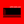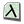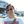0%
0%

# 1 - bit ttl / cmos finite state machine

a finite state machine based on the MC14500 Industrial control unit.

Similar projects worth following
Using EEPROMS an d-latches to create a 1-bit finite state machine that is
Turing complete. Based very much on the MC14500 Industrial control unit.

This is my CPU project, that was born out of trying to build 1 - bit combination logic ALU's from op-amps.  In doing the research for that project I determined that much of the MC14500 is a relatively simple (still a large number of transistors) finite state machine.    I decided to see if I could build a relatively fast state machine out of EEPROMS and D-Latches.

This project is very similar, and very much inspired by the UE14500 Vacuum tube computer Project.

My project goals are:

• Design the logic for the state machine I want to build (this is in it's first draft)
• Design / build / adapt? a work flow for simply making changes by either building or modifying existing tools for making binaries so that development is less cumbersome.
• Build a prototype on a breadboard to demonstrate a proof of concept using an 8-bit addressable d-latchs and some switches that can be used to compare 2 8 bit numbers and update a 8-bit display latch.
• Build a prototype, that is Turing-Complete with expanded functionality for moving 8bit data, jump / return and write a machine language monitor.
•

### 1-bit cpu (1 chip logic).circ

logism diagram for the logic which will be replaced by the EEPROM.

circ - 33.28 kB - 11/26/2021 at 03:38

• ### Contemplating 1-bit calculations, and challanges.

Dave Collins3 days ago 0 comments

The Changes to the Instruction set:

The MC14500 is primarily a 1-bit ICU.  and thus, its not generally meant to do arithmetic (though it can through manual bit manipulation, it's not very fast.)  Just as the UE14500 Project, our CPU will add a Add functions to add and subtract at a cost to some of the less useful functions.  Unlike the UE14500, we will take things a few steps further and add a external command register. This will allow for moving memory and register values around 8 bits at a time, this will be done by adding a OP, code to facilitate switching between the 1-bit state machine and the external command register (which will also be a finite state machine.)

Our modified instruction set:

Subtraction:

This CPU calculates data 1-bit at a time, though it actually looks at up to 3 bits to make a calculation, 1 bit from each register or the data bus, and a third carry bit stored from the previous operation.    The first challenge of this is there is no real way to do a full subtract operation (at least not 2's compliment, though a 1-bit subtractor is possible, it's not used here).  A full SBA (Subtraction by Addition) operation requires a XOR operation on the carry in bit, but only on the first operation.  In a multi-bit adding scenario this allows for adding a 1 at the same time as adding the compliment of the second operand (to subtract and get a 2's compliment value:

As you can see the adder will add 1, but invert the inputs on the B input this works simply enough for a multi-bit operation as 4 bits are calculated at once.  However this is slightly inconvenient when we try to do 1 bit operations.  Thisi is simply because we can no longer use the CI line as a mode selection for every bit.  The reason is simple; if we did that the adder would add 1 to each digit (this would not yield 2's compliment).  Instead our CPU will provide 2 operations for arithmetic; add and add compliment.

This approach is slightly more cumbersome as the CI register has to be preset with a carry in bit (but only once).  then each operation would add the compliment of the B register (when subtracting).  Additionally this adds more functionality to the MU (math unit) as now the add compliment operation can be used to add the inverse of the B register to zero (allowing us to put it's compliment in the results register).

Share

## Similar Projects

Project Owner Contributor

### Cambridge-1: a 4-bit 7400 series based CPUARITH-MATIC

Project Owner Contributor

### 16-bit CPU built in LogisimReed Foster

Project Owner Contributor

### Intel HEX files for FPGAs (no embedded CPUs!)zpekic

# Does this project spark your interest?

Become a member to follow this project and never miss any updates